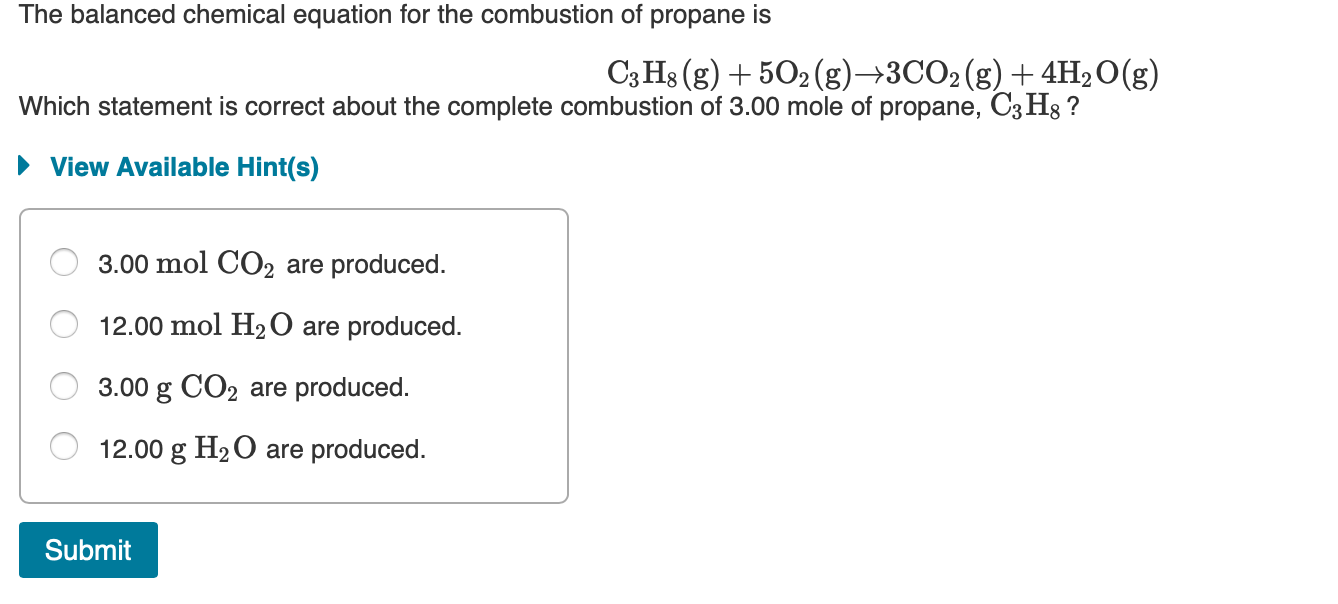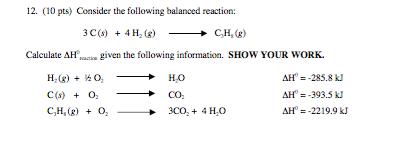Question

Calculate deltaHr for the reaction: C3H8(g) + 5O2(g)----->3CO2(g) + 4H2O(l)

Given:

3C(s) + 4H2(g)----->C3H8(g) deltaH -24.8 kcal

H2(g) + 1/2 O2(g)----->H2O(l) deltaH -68.3kcal

C(s) + O2(g)-------> CO2(g) deltaH -94.0 kcal

Let the reactions be numbered 1 to 4 from top to bottom

we need to find deltaHr for reaction 1

reaction 1 can be written as = - reaction 2 +4*reaction 3+ 3* reaction 4
so,
deltaHr (1) = -deltaHr (2) + 4*deltaHr (3) + 3*deltaHr (4)
= - (-24.8) + 4*(-68.3) + 3*(-94)
= 24.8 - 273.2 - 282
= -530.4 Kcal

#### Earn Coins

Coins can be redeemed for fabulous gifts.

Similar Homework Help Questions
• ### The combustion of propane, C3H8, occurs via the reaction C3H8(g)+5O2(g)→3CO2(g)+4H2O(g) with heat of formation values given...

The combustion of propane, C3H8, occurs via the reaction C3H8(g)+5O2(g)→3CO2(g)+4H2O(g) with heat of formation values given by the following table: Substance ΔH∘f (kJ/mol) C3H8 (g)=  -104.7 CO2(g)= −393.5 H2O(g)= −241.8 Calculate the enthalpy for the combustion of 1 mole of propane.

• ### The combustion of propane (C3H8) produces CO2 and H2O: C3H8 (g) + 5O2 (g) → 3CO2...

The combustion of propane (C3H8) produces CO2 and H2O: C3H8 (g) + 5O2 (g) → 3CO2 (g) + 4H2O (g) The reaction of 7.5 mol of O2 with 1.4 mol of C3H8 will produce ________ mol of CO2. Group of answer choices.

• ### Consider the following reaction: C3H8+5O2→3CO2+4H2OC3H8+5O2→3CO2+4H2O What is the reducing agent? C3H8C3H8 CC OO O2

Consider the following reaction: C3H8+5O2→3CO2+4H2OC3H8+5O2→3CO2+4H2O What is the reducing agent? C3H8C3H8 CC OO O2

• ### Consider the following equilibrium reaction: C3H8 (g) + 5O2 (g) -> 3CO2 (g) + 4 H2O...

Consider the following equilibrium reaction: C3H8 (g) + 5O2 (g) -> 3CO2 (g) + 4 H2O (l) delta H = -2220KJ if the amount of H2) is reduced to the system, the CO2 concentration increases. select one: a) Right b) False

• ### The balanced chemical equation for the combustion of propane is C3H8(g) + 5O2(g) +3CO2(g) + 4H2O(g)...The balanced chemical equation for the combustion of propane is C3H8(g) + 5O2(g) +3CO2(g) + 4H2O(g) Which statement is correct about the complete combustion of 3.00 mole of propane, C3Hg ? ► View Available Hint(s) O 3.00 mol CO2 are produced. O 12.00 mol H2O are produced. O 3.00 g CO2 are produced. O 12.00 g H2O are produced. Submit

• ### Consider the combustion of propane: C3H8 (g) + 5O2 (g) → 3CO2 (g) + 4H2O(l) ΔH...

Consider the combustion of propane: C3H8 (g) + 5O2 (g) → 3CO2 (g) + 4H2O(l) ΔH = –2221 kJ Assume that all of the heat comes from the combustion of propane. Calculate ΔH in which 5.00 g of propane is burned in excess oxygen at constant pressure.

• ### If we have C3H8 + 5O2 ---> 3CO2 + 4H2O a) How many liters of oxygen...

If we have C3H8 + 5O2 ---> 3CO2 + 4H2O a) How many liters of oxygen gas are required to react with 7.2 L of C3H8 if both gasses are at STP? b) How many grams of CO2 will be produced from 35 L C3H8 at 15 C and 1.65 atm? c) How many grams of water vapor can be produced when 15 L C3H8 at 15 C and 1.65 atm are reacted with 15 L O2 at -20.0 C...

• ### ﻿Propane (C3H8)burns according to the following balanced equation: C3H8(g)+5O2(g)→3CO2(g)+4H2O(g) Calculate ΔH∘rxnΔ for this reaction using standard...

﻿Propane (C3H8)burns according to the following balanced equation: C3H8(g)+5O2(g)→3CO2(g)+4H2O(g) Calculate ΔH∘rxnΔ for this reaction using standard enthalpies of formation. (The standard enthalpy of formation of gaseous propane is -103.9 kJ/molkJ/mol.) Express the enthalpy in kilojoules to four significant figures.

• ### Consider the following balanced reaction: 3C(s) +4H_2(g) rightarrow C3H8(g) Calculate given the following information. SHOW YOUR...Consider the following balanced reaction: 3C(s) +4H_2(g) rightarrow C3H8(g) Calculate given the following information. SHOW YOUR WORK. H_2(g)+v2o2 rightarrow HO deltaH0= -285.8 kJ C(s) + O2 rightarrow CO2 deltaH0 =-393.5 kJ C3H8(s) + O, rightarrow 3CO2+ 4H_20 deltaH0 = -2219.9 kJ

• ### calculate the enthalpy of the combustion of propane for your barbeque C3H8 + 5O2 -> 3CO2 + 4H2O propane -105 kJ...

calculate the enthalpy of the combustion of propane for your barbeque C3H8 + 5O2 -> 3CO2 + 4H2O propane -105 kJ/mol CO2 -394 kJ/mol Water -286 kJ/mol Ooxygen. 0